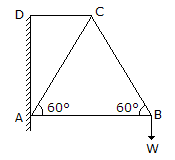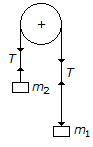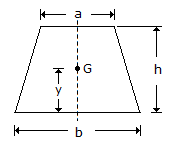Courses

# Test: Engineering Mechanics - 4

## 20 Questions MCQ Test Engineering Mechanics | Test: Engineering Mechanics - 4

Description
This mock test of Test: Engineering Mechanics - 4 for Mechanical Engineering helps you for every Mechanical Engineering entrance exam. This contains 20 Multiple Choice Questions for Mechanical Engineering Test: Engineering Mechanics - 4 (mcq) to study with solutions a complete question bank. The solved questions answers in this Test: Engineering Mechanics - 4 quiz give you a good mix of easy questions and tough questions. Mechanical Engineering students definitely take this Test: Engineering Mechanics - 4 exercise for a better result in the exam. You can find other Test: Engineering Mechanics - 4 extra questions, long questions & short questions for Mechanical Engineering on EduRev as well by searching above.
QUESTION: 1

Solution:
QUESTION: 2

Solution:
QUESTION: 3

### A lift moves downwards with an acceleration of 9.8 m/s2. The pressure exerted by a man on the floor of the lift is zero.

Solution:
QUESTION: 4

The maximum acceleration of a particle moving with simple harmonic motion is

Solution:
QUESTION: 5

Non-coplaner concurrent forces are those forces which

Solution:
QUESTION: 6

Which of the following statement is correct?

Solution:
QUESTION: 7

The maximum efficiency of a screw jack is

Solution:
QUESTION: 8

In a framed structure, as shown in the below figure, the force in the member AC is numerically equal to the force in member BC.Solution:
QUESTION: 9

Moment of inertia of a triangular section of base (b) and height (h) about an axis through its base, is

Solution:
QUESTION: 10

The force which acts along the radius of a circle and directed towards the centre of the circle is known as centripetal force.

Solution:
QUESTION: 11

Concurrent forces are those forces whose lines of action

Solution:
QUESTION: 12

According to the law of moments, if a number of coplaner forces acting on a particle are in equilibrium, then

Solution:
QUESTION: 13

If u1 and u2 are the velocities of two moving bodies in the same direction before impact and v1 and v2 are their velocities after impact, then coefficient of restitution is given by

Solution:
QUESTION: 14

Tension in the cable supporting a lift is more when the lift is moving __________ with an acceleration.

Solution:
QUESTION: 15

Two bodies of masses m1,and m2 are hung from the ends of a rope, passing oyer a frictionless pulley as shown in the figure below. The acceleration of the string will beSolution:
QUESTION: 16

The centre of gravity of a trapezium with parallel sides a and b lies at a distance of y from the base b, as shown in the below figure. The value of y isSolution:
QUESTION: 17

A machine having an efficiency less than 50%, is known as

Solution:
QUESTION: 18

The total momentum of a system of masses (i. e. moving bodies) in any one direction remains constant, unless acted upon by an external force in that direction. This statement is called

Solution:
QUESTION: 19

The velocity ratio of a differential pulley block with D and d as the diameters of larger and smaller pulley, is

Solution:
QUESTION: 20

The unit of mass moment of inertia in S.I. units is kg-m2.

Solution: